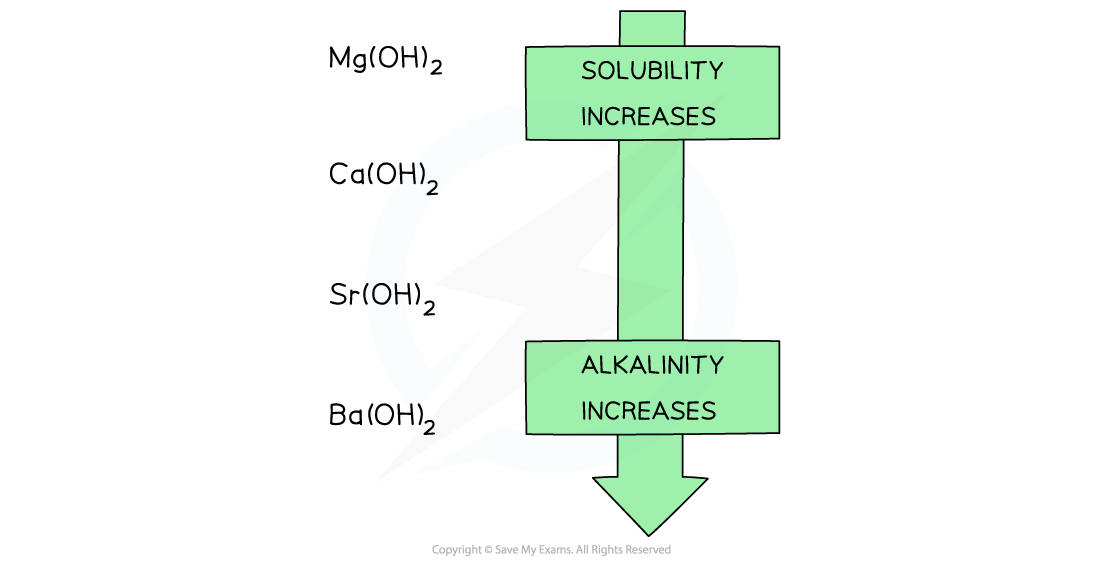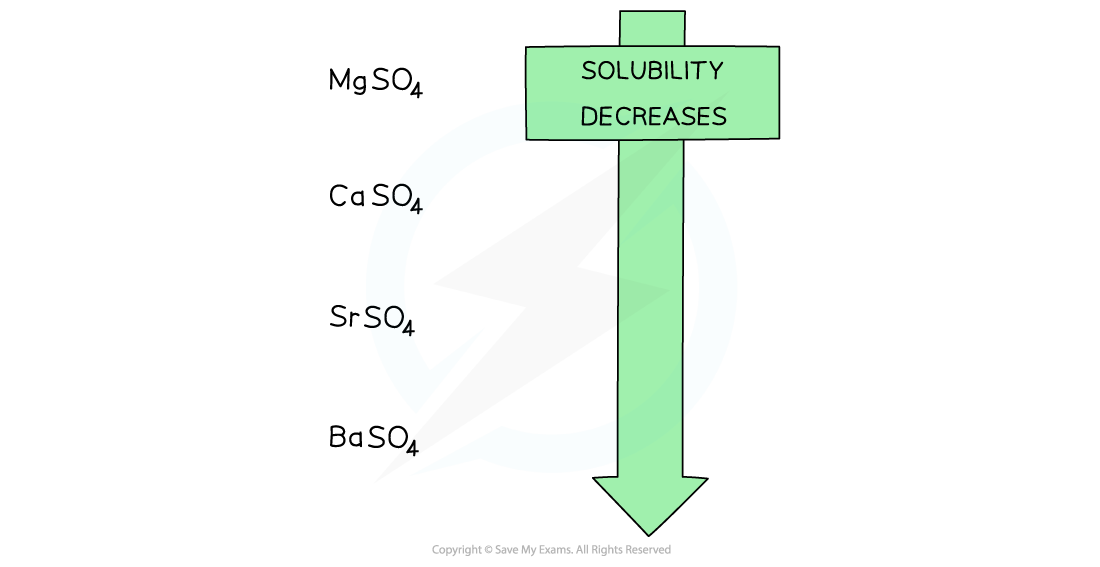# Edexcel A Level Chemistry:复习笔记2.2.3 Group 2 Hydroxides & Sulfates

### Group 2 Hydroxides & Sulfates

#### Group 2 hydroxides

• Going down the group, the solutions formed from the reaction of Group 2 oxides with water become more alkaline
• When the oxides are dissolved in water, the following ionic reaction takes place:

O2- (aq) + H2O(l) → 2OH- (aq)

• The higher the concentration of OH- ions formed, the more alkaline the solution
• The alkalinity of the formed solution can therefore be explained by the solubility of the Group 2 hydroxides

Solubility of the Group 2 hydroxides table• The hydroxides dissolve in water as follows:

X(OH)2 (aq) → X(aq) + 2OH- (aq)

Where X is the Group 2 element

• When the metal oxides react with water, a Group 2 hydroxide is formed
• Going down the group, the solubility of these hydroxides increases
• This means that the concentration of OH- ions increases, increasing the pH of the solution
• As a result, going down the group, the alkalinity of the solution formed increases when Group 2 oxides react with waterGoing down the group, the solubility of the hydroxides increases which means that the solutions formed from the reactions of the Group 2 metal oxides and water become more alkaline going down the group

#### Group 2 sulfates

• The solubility of the Group 2 sulfates decreasing going down the groupGoing down the group, the solubility of the sulfates decreases

#### Worked Example

Predicting properties of radium

Radium (Ra) is a radioactive element found below barium at the bottom of group 2Applying your knowledge of the group 2 elements, predict:

Property 1: The formula of the ion formed by Ra.

Property 2: The formulae of its oxide and hydroxide

Property 3: Its first ionisation energy

Property 4: Its reactivity compared with barium

Property 5: The relative pH of its saturated hydroxide solution compared with a saturated solution of calcium hydroxide

Property 6: The solubility of its sulfate compared to strontium sulfate

Property 7: The equation for the reaction of its solid oxide with dilute hydrochloric acid

Property 8: What would you expect to see if you mixed radium hydroxide solution with dilute sulfuric acid?

Property 1:

Since, Ra is in group 2, it will form an ion with +2 charge to give Ra2+

Property 2:

The group 2 oxides and hydroxides have general formula XO and X(OH)2 respectively where X is the group 2 element.

Therefore, radium oxide is RaO and radium hydroxide is Ra(OH)2

Property 3:

Radium is below barium so its atomic radius is larger than the atomic radius of barium.

This means that radium’s outermost electrons are even further away and are therefore even more easily removed than barium’s outermost electron pair.

The first ionization energy is between 450-480 kJ mol-1

Property 4:

Radium’s outermost electrons are even further away than in barium and are therefore more easily removed making radium more reactive than barium.

Property 5:

The group 2 hydroxides become more soluble going down the group.

Radium hydroxide will therefore be more soluble than calcium hydroxide. Since more hydroxide ions will be present in solution the pH should be higher than the pH of calcium hydroxide

Property 6:

The Group 2 sulfates become less soluble going down the group.

Radium sulfate will therefore be less soluble than strontium sulfate.

Property 7:

The general equation for the reaction of group 2 oxides with dilute hydrochloric acid is:

XO (s) + 2HCl (aq) → XCl2 (aq) + H2O (l)

The reaction of radium oxide with dilute hydrochloric acid is therefore:

RaO (s) + 2HCl (aq) → RaCl2 (aq) + H2O (l)

Property 8:

Radium sulfate will be formed in this reaction, however the solubility of group 2 sulfates decreases going down the group, therefore a white precipitate of radium sulfate will be formed in this reaction